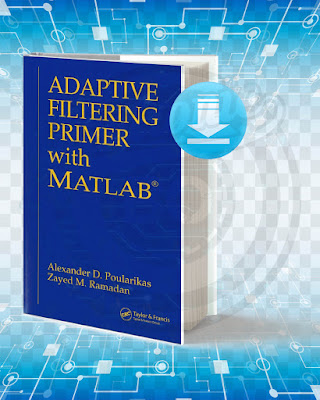## Adaptive Filtering Primer with MATLAB.

### Picture Of The Book:Adaptive Filtering Primer with MATLAB

Primer Filtering Adaptive Primer explains with MATLAB Adaptive Filter basics supported by many examples and computer simulations. It also offers many MATLAB and M functions along with computer experiments to illustrate how concepts apply to real-world problems. The book includes issues along with hints, suggestions, and solutions to resolve. An appendix to the matrix calculations complements the stand-alone coverage.

Title: Adaptive Filtering Primer with MATLAB.
Language: English.
Size: 2.64 Mb.
Pages: 202.
Format: Pdf.
Year: 2006.
Edition: 1.
Author: Alexander D. Poularikas Zayed M. Ramadan.

### Contents Of The Book:

Chapter 1: Introduction.
Chapter 2: Discrete-time signal processing.
Chapter 3: Random variables,sequences,and stochastic processes.
Chapter 4: Wiener filters.
Chapter 5: Eigenvalues of Rx properties of the error surface.
Chapter 6: Newton and steepest-descent method.
Chapter 7: The least-mean-square (LMS) algorithm.
Chapter 8: Variations of LMS algorithms.
Chapter 9: Least squares and recursive least-squares signal processing.15 sec

### The Best Free Books for Learning Electronics:

1.2.